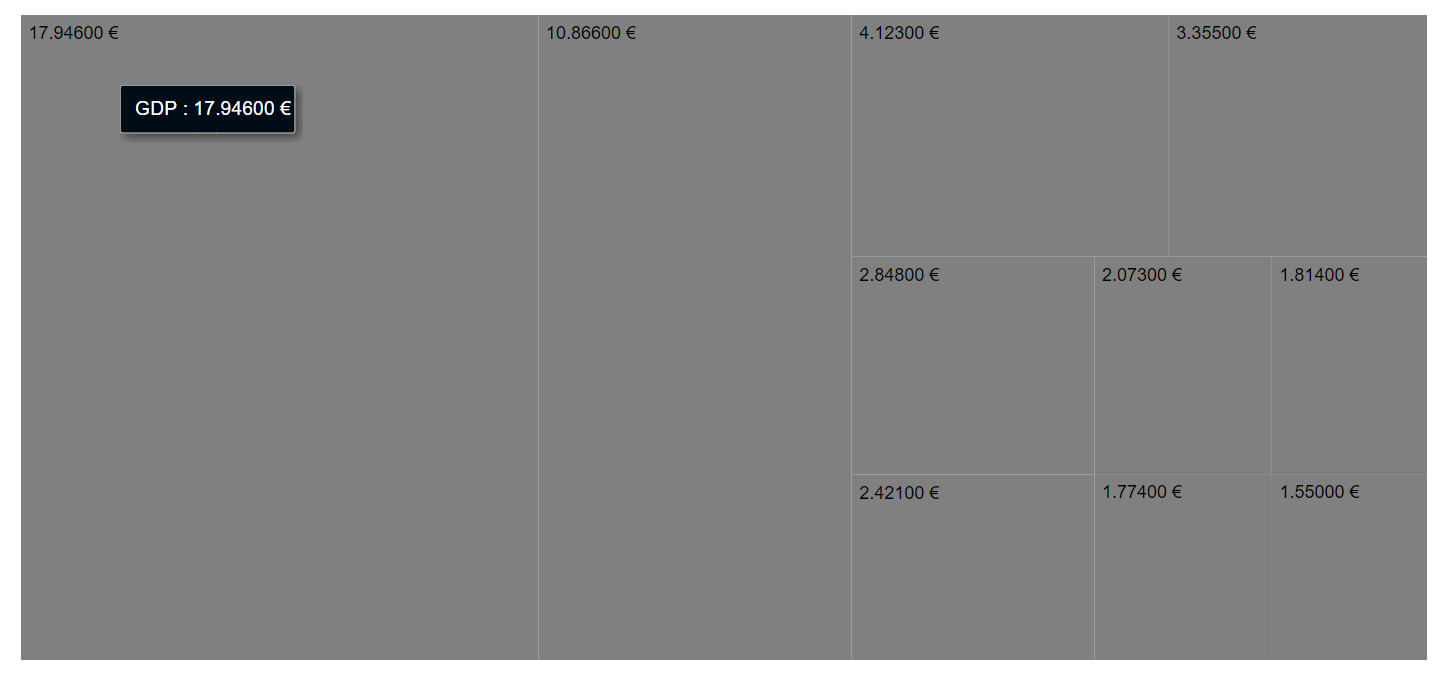Search results

# Globalization in Blazor TreeMap component

22 Jul 2021 / 2 minutes to read

The TreeMap component supports globalization for the following elements:

• Data Label
• Tooltip

Globalization is the process of designing and developing a component that works in different cultures or locales. The `Format` property is used to globalize number, date, and time values in the TreeMap component.

In the following code example, tooltip and Data Label is globalized to currency format in deutsch culture.

``````@using Syncfusion.Blazor.TreeMap

<SfTreeMap WeightValuePath="GDP" DataSource="GrowthReports" Format="C">
<TreeMapTooltipSettings Visible=true>
</TreeMapTooltipSettings>
</SfTreeMap>

@code {
public class GDPReport
{
public string State { get; set; }
public double GDP { get; set; }
public double Percentage { get; set; }
public int Rank { get; set; }
};
public List<GDPReport> GrowthReports = new List<GDPReport> {
new GDPReport {State="United States", GDP=17946, Percentage=11.08, Rank=1},
new GDPReport {State="China", GDP=10866, Percentage= 28.42, Rank=2},
new GDPReport {State="Japan", GDP=4123, Percentage=-30.78, Rank=3},
new GDPReport {State="Germany", GDP=3355, Percentage=-5.19, Rank=4},
new GDPReport {State="United Kingdom", GDP=2848, Percentage=8.28, Rank=5},
new GDPReport {State="France", GDP=2421, Percentage=-9.69, Rank=6},
new GDPReport {State="India", GDP=2073, Percentage=13.65, Rank=7},
new GDPReport {State="Italy", GDP=1814, Percentage=-12.45, Rank=8},
new GDPReport {State="Brazil", GDP=1774, Percentage=-27.88, Rank=9},
new GDPReport {State="Canada", GDP=1550, Percentage=-15.02, Rank=10}
};
}``````

Refer `here` to configure localization for the Blazor server application, and `here` for the Blazor web assembly application.

On successful configuration of localization the TreeMap will be rendered, as following.